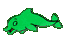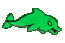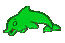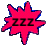Algebra Help - Polynomial Help - Multiply Binomial times Binomial - Game Tips:

- In the example (a+b)(c-d) you distribute (multiply) 'a' times (c-d) to get 'ac-ad' and then
you distribute '+b' times (c-d) to get '+bc-bd'. So the answer to (a+b)(c-d)= ac-ad+bc-bd.
- You finish each question by adding or subtracting any 'like terms'.
For example, (a+3)(a+2) = a2+2a+3a+6 when finished, becomes a2+5a+6 .

- Your Game Score is reduced by the number of dolphin hits.

- To slow the game speed repeat tap/click on the word Slider.
- To increase the game speed repeat tap/click on the word Math.
- Speed can also be adjusted with a keyboard's - and + keys.

- Refresh/Reload the web page to restart the game.